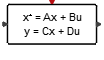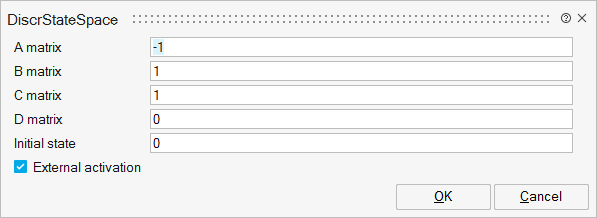# DiscrStateSpace

This block implements a discrete-time linear state-space system when activated at a fixed rate. The system is defined by the A,B,C,D matrices and the initial state x0.Dynamical

## Description

This block implements a discrete-time linear state-space system when activated at a fixed rate.

The system is defined by the A,B,C,D matrices and the initial state vector x0.

The system is xdot = Ax + bu and y = Cx + du where x (size n) is the vector of the state variables, u is the vector of inputs (size m) and y (size r) is the vector of outputs.

## ParametersNameLabelDescriptionData TypeValid Values

A

A matrix

real nxn matrix where n is the number of states, default value: 1.

Matrix

B

B matrix

real nxm matrix where n is the number of states and m is the number of inputs, default value: 1. B=[ ] if block has no input.

Matrix

C

C matrix

real rxn matrix, default value: 1. r is the number of outputs and n is the number of states.

Matrix

D

D matrix

real rxm matrix where r is the number of outputs and m is the number of inputs, default value: 1.

Matrix

x0

Initial state

vector of size n or scalar (expanded to a vector of size n).

Vector

externalActivation

External activation

Number

0
1

## Ports

NameTypeDescriptionIO TypeNumber

Port 1

explicit

output

1

Port 2

explicit

input

1

Port 3

activation

input

externalActivation

NameValueDescription

always active

no

direct-feedthrough

no, except when D is not zero

zero-crossing

no

mode

no

continuous-time state

no

discrete-time state

yes